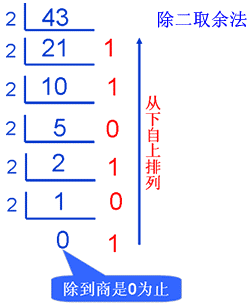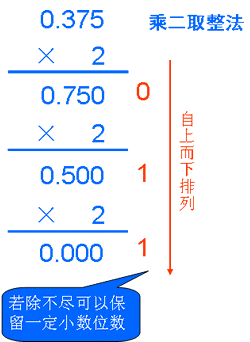while(true) rp++;

# 一、计算机基础

## TCP/IP协议

• 链路层：这是 TCP/IP 结构的第一层，也叫网络接口层，其功能是提供网 络相邻节点间的信息传输以及网络硬件和设备驱动。
• 网络层：（IP 协议层）其功能是提供源节点和目的节点之间的信息传输服 务，包括寻址和路由器选择等功能。
• 传输屋：（TCP 协议）其功能是提供网络上的各应用程序之间的通信服务。
• 应用层：这是 TCP/IP 最高层，其功能是为用户提供访问网络环境的手段， 主要提供 FTP、TELNET、GOPHER 等功能软件。 IP 协议适用于所有类型网络。TCP 协议则处理 IP 协议所遗留的通信问题，为应用程序提供 可靠的通信连接，并能自动适应网络的变化。TCP/IP 目前成为最为成功的网络体系结构和 协议规范。

## IPV4

• 第一段取值在 1～127 之间，表示主机所在的网络属于大型网（A 类网），其值就是网络的网络号，后三段数字表示该主机号；
• 第一段数字取值在 128～191 之间，表示主机所在网络为 中型网（B 类网），第一段和第二段的数字联合表示该网络的网络号，第三段数字则表示子 网号，第四段则是该主机号；
• 第一段数字取值为 192～223 的，表示该主机所在的网络为小 型网（C 类网），第一、二、三段数字的组合表示该网络的网络号，第四段是主机号。连接 到Internet上的计算机就是通过这些 32 位的 IP 地址相互联系的。

## IPV6

IPv6的地址长度为128bit，是IPv4地址长度的4倍。于是IPv4点分十进制格式不再适用，采用十六进制表示。

## 比特，字节和其他单位

$8BIT=1BYTE$ $1024B=1KB$ $1024KB=1MB$

## 机器语言，汇编语言和高级语言

• 机器语言是用二进制代码表示的计算机能直接识别和执行的一种机器指令的集合。它是计算 机的设计者通过计算机的硬件结构赋予计算机的操作功能。机器语言具有灵活、直接执行和 速度快等特点。不同型号的计算机其机器语言是不相通的，按着一种计算机的机器指令编制的程序，不能在另一种计算机上执行
• 虽然机器语言、汇编语言是低级语言，但是，用汇编语言、机器语言来编制、运行软件时，占用内存空间少，速度快，具有高级语言不可替代的用处。
• 语言相近并为计算机所接受和执行的计算机语言称高级语言。高级语言是面向用户的语言。 无论何种机型的计算机，只要配备上相应的高级语言的编译或解释程序，则用该高级语言编写的程序就可以通用。BASIC、Python使用解释方式执行，PASCAL、C使用编译方式执行

• BASIC语言全称是 Beginner’s all Purpose Symbolic Instruction Code，意为“初学者通用符号指令代码“。
• PASCAL是一种结构程序设计语言，由瑞士苏黎世联邦工业大学的沃斯(N.Wirth)教授研制，于1971年正式发表。是从ALGOL60衍生的，但功能更强且容易使用。
• C语言是美国AT&T（电报与电话）公司为了实现UNIX系统的设计思想而发展起来的语言工具。C语言的主要特色是兼顾了高级语言和汇编语言的特点，简洁、丰富、可移植。
• C++是Bell实验室的Bjame Sgoustrup研发的，他最初将C改良为带类的C（C with classes），后来正式命名为C++。

## 其它杂项

• RAM即内存，这种存储器在断电时将丢失其存储内容，故主要用于存储短时间使用的程序。
• ROM即硬盘，断电时不会丢失其储存数据，是一种只能读出事先所存数据的固态半导体存储器。

Intel 8086Intel首个16位处理器。

C++不是历史上第一个支持面向对象的语言，Simula 67被认为是最早的面向对象程序设计语言

1944年，美籍匈牙利数学家冯·诺依曼提出计算机基本结构和工作方式的设想，为计算机的诞生和发展提供了理论基础。时至今日，尽管计算机软硬件技术飞速发展，但计算机本身的体系结构并没有明显的突破，当今的计算机仍属于冯·诺依曼架构。 其理论要点如下：

1、计算机硬件设备由存储器、运算器、控制器、输入设备和输出设备5部分组成。

2、存储程序思想——把计算过程描述为由许多命令按一定顺序组成的程序，然后把程序和数据一起输入计算机，计算机对已存入的程序和数据处理后，输出结果。

# 二、进制转换

## 十进制转R进制：

$(173)10 = (10101101)2$ ，需要说明的是10进制转其它进制时， 整数部分短除法后结果从下到上取，小数部分短除法后结果从上到下取。• 重点： (173.375)10 = (AD.6)16 $，需要说明的是**每次转换整数部分时，所记下的是余数，用于继续计算的是商。** # 三、逻辑运算 运算符：**非：not ¬  与：and ∧  或：or ∨ 异或：xor ⊕** 运算级比较： **非 > 与 > 或 、异或**，因此遇到一个表达式时，先处理¬，再处理∧，最后才轮到∨。 - **异或**：二元运算符：a⊕b **[如果a和b同为一种状态，则结果是false；否则是true]** 表格如下： > | a | b | answer | | :---: | :---: | :---: | | true | true | false | | true | false | true | | false | true | true | | false | false | false | - **或**：二元运算符：a∨b **[如果a和b中有true，则结果是true；否则是false]** - **与**：二元运算符：a∧b **[如果a和b都是true，则结果是true；否则是false]** - **非**：单目运算符：¬a **[对于a取反]** # 四、原码，反码，补码 计算机中要处理的数分无符号数和有符号数两种。有符号数在计算机中用“0”表示正数，“1”表示负数。 - 原码：最高位用“0”表示正数，“1”表示负数，其它位为无符号数，用这种方法表示的数称为原码。用 这种数进行两个异号数相加或两个同号数相减时很不方便。为了将减法运算转 换为加法运算，需要引入反码和补码的概念。 - 反码：对于正数：反码＝原码；对于负数：除符号位外，其他各位分别 0,1 取反。 > 例：原码 01000101，其补码为 01000101 原码 11000101，其补码为 10111010。 - 补码：正数的补码＝原码，对于负数：补码＝反码＋1。 > 例：01000101 的补码为 01000101，11000101 的补码为 10111011。 总之：**正数的原码＝反码＝补码。负数的补码＝反码＋1。负数的反码是原码除符号位外，其他各位分别 0,1 取反后得到的结果。** # 五、图的基础知识 ## 有/无向图 - 如果给图的每条边规定一个方向，那么得到的图称为**有向图**。在有向图中，与一个节点相关联的边有出边和入边之分。 - 边没有方向的图称为**无向图**。 ## 度 - **在无向图中，每个节点连边的条数就是该节点的度数。** - 在有向图中，指向该节点的边数称为入度；反之，则称为出度；**在有向图中，度的大小等于某点出入度之和。** - **在树中，该节点的子女的个数称为节点的度。** ## 最小生成树 一个有 n 个结点的连通图的生成树是原图的极小连通子图，且包含原图中的所有 n 个结点，并且有保持图连通的最少的边。 最小生成树可以用kruskal（克鲁斯卡尔）算法或prim（普里姆）算法求出。 ## 图的储存 - 邻接矩阵：用一个一维数组存放图中所有顶点数据；用一个二维数组存放顶点间关系（边或弧）的数据，这个二维数组称为**邻接矩阵**。邻接矩阵又分为有向图邻接矩阵和无向图邻接矩阵。 - 邻接表：图的邻接表存储方法跟树的孩子链表示法相类似，是一种顺序分配和链式分配相结合的存储结构。如这个表头结点所对应的顶点存在相邻顶点，则把相邻顶点依次存放于表头结点所指向的单向链表中。如词条概念图所示，表结点存放的是邻接顶点在数组中的索引。*对于无向图来说，使用邻接表进行存储也会出现数据冗余，表头结点A所指链表中存在一个指向C的表结点的同时，表头结点C所指链表也会存在一个指向A的表结点。* # 六、树 ## 树的基本概念 树状图是一种数据结构，它是由n（n>=0）个有限结点组成一个具有层次关系的集合。把它叫做“树”是因为它看起来像一棵倒挂的树，也就是说它是根朝上，而叶朝下的。它具有以下的特点： - 每个结点有零个或多个子结点； - 没有父结点的结点称为根结点； - 每一个非根结点有且只有一个父结点； - 除了根结点外，每个子结点可以分为多个不相交的子树。 ## 基本术语 - 节点的度：一个节点含有的子树的个数称为该节点的度； - 叶节点或终端节点：度为0的节点称为叶节点； - 非终端节点或分支节点：度不为0的节点； - 双亲节点或父节点：若一个节点含有子节点，则这个节点称为其子节点的父节点； - 孩子节点或子节点：一个节点含有的子树的根节点称为该节点的子节点； - 兄弟节点：具有相同父节点的节点互称为兄弟节点； - 树的度：一棵树中，最大的节点的度称为树的度（通常是指根节点的度）； - 节点的层次：从根开始定义起，根为第1层，根的子节点为第2层，以此类推； - 树的高度或深度：树中节点的最大层次； - 堂兄弟节点：双亲在同一层的节点互为堂兄弟； - 节点的祖先：从根到该节点所经分支上的所有节点； - 子孙：以某节点为根的子树中任一节点都称为该节点的子孙。 - 森林：由m（m>=0）棵互不相交的树的集合称为森林； ## 二叉树 在计算机科学中，**二叉树是每个结点最多有两个子树的树结构。**通常子树被称作“左子树”（left subtree）和“右子树”（right subtree）。 ## 特殊类型二叉树 - 完全二叉树：**除了最下层，其他每层都饱满，最下层的结点都集中在该层最左边的若干位置上。** - 满二叉树：**每层都饱满。** - 二叉排序树：**二叉排序树**（又称二叉查找树）或者是一棵空树，或者是具有下列性质的二叉树： - 若左子树不空，则左子树上所有结点的值均小于它的根结点的值； - 若右子树不空，则右子树上所有结点的值均大于它的根结点的值； - 左、右子树也分别为二叉排序树。 ## 二叉树遍历 所谓先序、中序、后序，实则指的是：**对于每一棵子树，把子树的根节点放在怎么样的地位上**。 - 先序遍历：**根左右** - 中序遍历：**左根右** - 后序遍历：**左右根** **已知先序和中序，二叉树是唯一的。已知后序和中序，二叉树是唯一的。已知先序和后序，二叉树不是唯一的。** ## 二叉树注意事项 - 不论二叉树有多少个结点，最少都是只有1个叶节点。 - 二叉树每层所能容纳的数量呈2的次方增长：1，2，4，8，16…… ## 堆 堆是一种特殊的二叉树。 - 当堆中的最大元素存放在根结点中，且每一结点的子树中的结点值都小于等于该结点的值时，这种堆是**“大根堆”**； - 反之，对除根以外的每个结点i，A[parent(i)]≤A[i]的堆，称为**“小根堆”**。 # 七、排序算法 ## 排序算法时间复杂度 | 排序算法 | *平均*时间复杂度 | | -----------: | -----------: | | 冒泡排序 |$ O(n^2) $| | 选择排序 |$ O(n^2) $| | 插入排序 |$ O(n^2) $| | 快速排序 |$ O(nlogn) $| | 归并排序 |$ O(nlogn) $| ## 排序算法稳定性 **堆排序、快速排序、希尔排序、选择排序是不稳定的排序算法；** **基数排序、冒泡排序、插入排序、归并排序是稳定的排序算法。** 排序算法的稳定性通俗地讲就是能保证排序前2个相等的数其在序列的前后位置顺序和排序后它们两个的前后位置顺序相同。在简单形式化一下，如果Ai = Aj， Ai原来在位置前，排序后Ai还是要在Aj位置前。 # 八、字符串 ## 子串 字符串中**任意个连续的字符组成的子序列**称为该串的子串。 注意： - **连续**； - **空串属于子串**； - **两个子串如果内容相同则只算一个**。 ## 表达式类型 - **中缀表达式**就是常见的运算表达式，如：(3+4)×5-6 - **前缀表达式**又称**波兰式**，前缀表达式的运算符位于操作数之前，如：- × + 3 4 5 6 - **后缀表达式**又称**逆波兰表达式**,与前缀表达式相似，只是运算符位于操作数之后，如：3 4 + 5 × 6 - ### **中缀表达式**转换成**后缀表达式** > a + b * c - (d + e) > > 按照运算符的优先级对所有的运算单位加括号。 ((a + (b * c)) - (d + e)) > > 转换中缀与后缀表达式后缀：把运算符号移动到对应的括号后面。 ((a (b c) * ) + (d e) + ) - > > 把括号去掉，记得到了后缀表达式： a b c * + d e + - # 九、排列组合 ## 排列 **n个不同元素中，任取m个元素，按照一定的顺序排成一列**，叫做从n个不同元素中取出m个元素的一个排列。 >- 公式：$ A(m,n)=n(n-1)(n-2)...(n-m+1)=n! / (n-m)! $## 组合 **n个不同元素中，任取m个元素，并成一组**，叫做从n个不同元素中取出m个元素的一个组合。 >- 公式：$ C(m,n)=A(m,n)/A(m,m) $>- 也即：$ C(m,n)=n(n-1)(n-2)...(n-m+1)/m!=n! / m! (n-m)! $## Catalan数列 ![Catalan](https://img.grouplus.com/admin-files/23410/ueditor_image/zc_20170923115134798) ### Catalan数在组合计算中的应用： - 一个栈(无穷大)的进栈序列为1，2，3，…，n，有多少个不同的出栈序列？ - 3n个节点构成的二叉树，共有多少种情形？ ## 圆排列 n个人全部来围成一圈为Q(n,n)，其中已经排好的一圈，从不同位置断开，又变成不同的队列。所以：$ Q(n,n)n=P(n,n) >>> Q(n)=P(n,n)/n=(n-1)! $由此可知，部分圆排列：$ Q(n,r)＝P(n,r)/r=n!/(r*(n-r)!). \$

# 十、常用模板

## 高精度

#include<iostream>
#include<cstdio>
#include<string>
#include<algorithm>
#define MAX_NUM 10001
using namespace std;
void GaoJinJiaFa(string sa,string sb);
void GaoJinJianFa(string sa,string sb);
void GaoJinChengFa(string sa,string sb);
/*
重要提示：千万不要试图越出数组界求元素值！
int array[MAX_NUM]只能让你索引array~array[MAX_NUM-1]。
*/
int main(){
string sa,sb;
cin>>sa>>sb;
cout<<"+: ";
GaoJinJiaFa(sa,sb);
cout<<endl<<"-: ";
GaoJinJianFa(sa,sb);
cout<<endl<<"*: ";
GaoJinChengFa(sa,sb);
cout<<endl;
return 0;
}

//高精度加法
void GaoJinJiaFa(string sa,string sb){
//init
int a[MAX_NUM],b[MAX_NUM],c[MAX_NUM],maxl=max(sa.length(),sb.length());
for(int i=0;i<MAX_NUM;i++) a[i]=0,b[i]=0,c[i]=0;
reverse(sa.begin(),sa.end());
reverse(sb.begin(),sb.end());
for(int i=0;i<(int)sa.length();i++) a[i]=sa[i]-'0';
for(int i=0;i<(int)sb.length();i++) b[i]=sb[i]-'0';

//calc
for(int i=0;i<maxl;i++){
c[i]+=a[i]+b[i];
if(c[i]>=10){
c[i+1]++;
c[i]-=10;
}
}

//print
bool isMeaninglessZero=true;
for(int i=MAX_NUM-1;i>=0;i--){
if(isMeaninglessZero){
if(c[i]==0) continue;
else isMeaninglessZero=false;
}
cout<<c[i];
}
}
/*要点：
1、开始时反转，方便之后进位
2、要用“c[i]+=a[i]+b[i]”而不是“c[i]=a[i]+b[i]”，来保存进位
3、结束时反转，正序输出
*/

//高精度减法
void GaoJinJianFa(string sa,string sb){
//init
if((int)sa.length()<(int)sb.length()||((int)sa.length()==(int)sb.length()&&sa<sb)) swap(sa,sb);///全部大减小处理
int a[MAX_NUM],b[MAX_NUM],c[MAX_NUM],maxl=max(sa.length(),sb.length());
for(int i=0;i<MAX_NUM;i++) a[i]=0,b[i]=0,c[i]=0;
reverse(sa.begin(),sa.end());
reverse(sb.begin(),sb.end());
for(int i=0;i<(int)sa.length();i++) a[i]=sa[i]-'0';
for(int i=0;i<(int)sb.length();i++) b[i]=sb[i]-'0';

//calc
for(int i=0;i<maxl;i++){
c[i]+=a[i]-b[i];
if(c[i]<0){
c[i]+=10;
c[i+1]--;
}
}

//print
bool isMeaninglessZero=true;
for(int i=MAX_NUM-1;i>=0;i--){
if(isMeaninglessZero){
if(c[i]==0) continue;
else isMeaninglessZero=false;
}
cout<<c[i];
}
if(isMeaninglessZero) cout<<0;///如果结果是0，还得再输出它
}
/*要点：
1、开始时反转，方便之后退位
2、要用“c[i]+=a[i]-b[i]”而不是“c[i]=a[i]-b[i]”，来保存退位
3、结束时反转，正序输出
*/

//高精度乘法
void GaoJinChengFa(string sa,string sb){
//init
int a[MAX_NUM],b[MAX_NUM],c[MAX_NUM];
for(int i=0;i<MAX_NUM;i++) a[i]=0,b[i]=0,c[i]=0;
reverse(sa.begin(),sa.end());
reverse(sb.begin(),sb.end());
for(int i=0;i<(int)sa.length();i++) a[i]=sa[i]-'0';
for(int i=0;i<(int)sb.length();i++) b[i]=sb[i]-'0';

//calc
for(int i=0;i<(int)sa.length();i++){
for(int j=0;j<(int)sb.length();j++){
c[i+j]+=a[i]*b[j];
if(c[i+j]>=10){
c[i+j+1]+=c[i+j]/10;
c[i+j]%=10;
}
}
}

//print
bool isMeaninglessZero=true;
for(int i=MAX_NUM-1;i>=0;i--){
if(isMeaninglessZero){
if(c[i]==0) continue;
else isMeaninglessZero=false;
}
cout<<c[i];
}
}
/*要点：
1、开始时反转，方便之后进位
2、a的第i位、b的第j位（从0开始计量位数时）的结果在c的第i+j位。
3、要用“c[i+j]+=a[i]*b[i]”而不是“c[i+j]=a[i]*b[i]”，来保存进位
4、结束时反转，正序输出
*/

## 快速幂

#include<bits/stdc++.h>
using namespace std;
int main(void){
int a,b;
cin>>a>>b;
if(b==0){
cout<<1;
return 0;
}//特判
long long ans=1,t=a;
while(b>0){
if(b%2==1) ans*=t;//只增一次
t=t*t;//次方数翻倍
b/=2;
}//快速幂
cout<<ans;
return 0;
}

## 排序

#include<iostream>
#include<cstdio>
#include<algorithm>
using namespace std;
int n,l,wayTo;

bool cmp(int a,int b){return a<b;}//cmp的要点：从小到大排用<，从大到小用>。
void STLPaiXu(){
sort(l,l+n,cmp);
for(int i=0;i<n;i++) cout<<l[i]<<' ';
}//STL排序

void TongPaiXu(){
int bucket;
for(int i=0;i<100001;i++) bucket[i]=0;
for(int i=0;i<n;i++) bucket[l[i]]++;//如果有这个数，就在桶中做记号
for(int i=0;i<100001;i++){
if(bucket[i]){
for(int j=0;j<bucket[i];j++) cout<<i<<' ';
}
}
}//桶排序

void MaoPaoPaiXu(){
for(int i=0;i<n;i++){
for(int j=i+1;j<n;j++){
if(l[j]<l[i]) swap(l[j],l[i]);
}
}//如果一个数比后面的某个数大，则互换
for(int i=0;i<n;i++) cout<<l[i]<<' ';
}//冒泡排序

void ChaRuPaiXu(){
for(int i=0;i<n;i++){
for(int j=i;l[j]<l[j-1];j--) swap(l[j],l[j-1]);
}//如果一个数比前面的那个数小，则互换，和冒泡排序类似
for(int i=0;i<n;i++) cout<<l[i]<<' ';
}//插入排序

void quick_sort(int left,int right){
if(left<right){
int i=left,j=right,x=l[i];
while(i<j){
if(l[j]>=x&&i<j) j--;//从右边寻找第一个比基准数小的数
if(l[i]<=x&&i<j) i++;//从左边寻找第一个比基准数大的数
swap(l[i],l[j]);//交换
}
swap(l[left],l[i]);//将基准数和第一个比基准数小的数交换（现在那个数是l[i]）
quick_sort(left,i-1);
quick_sort(i+1,right);//二分深入
}
}
void KuaiSuPaiXu(){
quick_sort(0,n-1);
for(int i=0;i<n;i++) cout<<l[i]<<' ';
}//快速排序

int main(){
cout<<"Which way are you going to use?\n";
cin>>wayTo;
cout<<"How many numbers are you going to give?\n";
cin>>n;
for(int i=0;i<n;i++) scanf("%d",&l[i]);
switch(wayTo){
case 0:STLPaiXu();break;
case 1:TongPaiXu();break;
case 2:MaoPaoPaiXu();break;
case 3:ChaRuPaiXu();break;
case 4:KuaiSuPaiXu();break;
}
return 0;
}

## 合并排序

#include<iostream>
#include<cstdio>
#include<algorithm>
using namespace std;
int n,a,temp;
//将first-mid，mid-last两个有序数列合并
void mergeArray(int first,int mid,int last){
int i=first,j=mid+1;
int m=mid,n=last,k=0;//k为临时变量
while(i<=m&&j<=n){//两段序列
if(a[i]<=a[j]) temp[k++]=a[i++];
else temp[k++]=a[j++];
}
while(i<=m) temp[k++]=a[i++];
while(j<=n) temp[k++]=a[j++];
for(int i=0;i<k;i++)
a[first+i]=temp[i];//用了temp数组来保存有序化的序列，最后再还原回去
}
/*比较二个数列的第一个数，谁小就先取谁，

void mergeSort(int first,int last){
if(first<last){//如果还没有分解成最小份
int mid=(first+last)/2;
mergeSort(first,mid);
mergeSort(mid+1,last);//二分，分别去排序
mergeArray(first,mid,last);//合并有序数组
}
}
int main(){
cin>>n;
for(int i=0;i<n;i++) cin>>a[i];
mergeSort(0,n-1);
for(int i=0;i<n;i++) cout<<a[i]<<' ';
return 0;
}

## 埃氏筛质数

#include<bits/stdc++.h>
using namespace std;
int p,m;
int main(void){
cin>>m;
for(int i=2;i<=m;i++){
if(p[i]==0){
for(int j=i*2;j<=m;j+=i){
p[j]=1;
}
}
}//如果一个数是质数的倍数，则它不是质数，否则是
if(m==0||m==1) cout<<"false";
else if(p[m]) cout<<"false";
else cout<<"true";
return 0;
}

## 欧拉筛质数

#include<bits/stdc++.h>
using namespace std;
int m,isprime,primelist,cnt=0;
int main(void){
cin>>m;
for(int i=2;i<=m;i++){
if(isprime[i]==0) primelist[cnt++]=i;
for(int j=0;j<cnt&&primelist[j]*i<=m;j++){
isprime[primelist[j]*i]=1;//质数的倍数是和数
if(i%primelist[j]==0) break;//避免重复计算
}
}
if(isprime[m]||m==0||m==1) cout<<"false\n";
else cout<<"true\n";
return 0;
}

## 求最大公因数

#include<iostream>
#include<cstdio>
using namespace std;
int gcd(int m,int n){
if(n==0) return m;//当上一次m%n==0时，意味着已经寻到了最大公因数
return gcd(n,m%n);//m此处大于n；(m%n)小于n
}//gcd: 最大公因数，辗转相除法
int main(void){
int a,b;
cin>>a>>b;
if(a<b) swap(a,b);
cout<<gcd(a,b);
return 0;
}

## 并查集

#include<iostream>
#include<cstdio>
#include<algorithm>
using namespace std;
int n,m,f,x,y,z;
int finder(int k){
if(f[k]==k) return k;//如果已经查到祖宗节点了，返回该节点
else return f[k]=finder(f[k]);//路径压缩，即把所有节点的祖先直接定为最高的祖先
}
int main(){
scanf("%d %d",&n,&m);
for(int i=1;i<=n;i++) f[i]=i;//最开始将每个数的父节点设为自己本身
for(int i=0;i<m;i++){
scanf("%d %d %d",&z,&x,&y);
if(z==1) f[finder(x)]=finder(y);//让x所在集合变成y所在集合的子集（让x的祖宗节点更换为y的祖宗节点）
else{
if(finder(x)==finder(y)) cout<<"Y\n";//如果二者回溯回去，拥有相同的祖宗节点，则在同一个节点中
else cout<<"N\n";//否则不在同一个集合中
}
}
return 0;
}
/*并查集简介：

（即靠左，靠右也是同一原理）。
*/